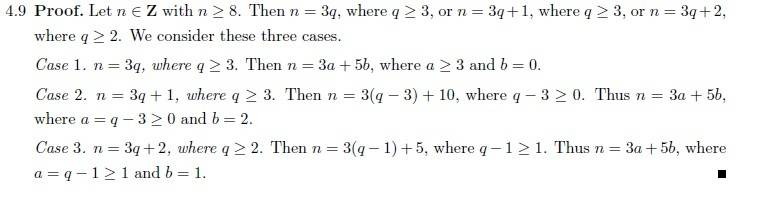# What's going on in this proof about integers?

## Homework Statement

Prove that for every integer n>=8, there exists nonnegative integers a and b, such that n =3a+5b

## The Attempt at a Solution

I'm trying to understand the proof of this. It goes as follows:I am having a hard time figuring out what is going on in any of the three cases.

Firstly, why did they pick 3q, 3q+1, and 3q+2?
They could do this proof using n = 5q, 5q+1, 5q+2, 5q+3, 5q+4 also correct? It would just have more cases, and therefor, be more work?

Then, about the cases.

Case I: n = 3q
I see what they did here. Since they picked to represent their integer as 3q, they supposed that b = 0, and then it's easy to show there exists an a, since a = q at that point. And a >=3 because n = 3q needs to be greater than 8.

Case II: n = 3q+1
Here is where I am troubled.
How do they make the jump to claim that n = 3(q − 3) + 10 ??
What is the thought process behind this?

I would appreciate any explanation that you could provide. Thanks!

Dick
Science Advisor
Homework Helper

## Homework Statement

Prove that for every integer n>=8, there exists nonnegative integers a and b, such that n =3a+5b

## The Attempt at a Solution

I'm trying to understand the proof of this. It goes as follows:I am having a hard time figuring out what is going on in any of the three cases.

Firstly, why did they pick 3q, 3q+1, and 3q+2?
They could do this proof using n = 5q, 5q+1, 5q+2, 5q+3, 5q+4 also correct? It would just have more cases, and therefor, be more work?

Then, about the cases.

Case I: n = 3q
I see what they did here. Since they picked to represent their integer as 3q, they supposed that b = 0, and then it's easy to show there exists an a, since a = q at that point. And a >=3 because n = 3q needs to be greater than 8.

Case II: n = 3q+1
Here is where I am troubled.
How do they make the jump to claim that n = 3(q − 3) + 10 ??
What is the thought process behind this?

I would appreciate any explanation that you could provide. Thanks!

n=3q+1, 3(q-3)+10=3q-9+10=3q+1=n. It's not as big a jump as you think.

•1 person
Ah I see. So they are still playing the make one side look like the other game. Thanks again!

Dick
Science Advisor
Homework Helper
Ah I see. So they are still playing the make one side look like the other game. Thanks again!

That's a good way to phrase it. They are just splitting up into something divisible by 3 plus something divisible by 5.

Yeah.

That "add 0 to an equation by adding some quantity x and then subtracting x again" thing always catches me off guard. I'm very familiar with that trick from various calc II integrals,

but it still always hits me like a truck.